Gaussian Elimination with Partial Pivoting

Terry D. Johnson
10.001 Fall 2000

In the problem below, we have order of magnitude differences between coefficients in the different rows.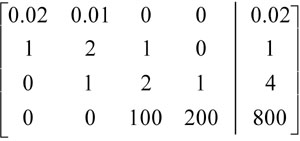Step 0a: Find the entry in the left column with the largest absolute value. This entry is called the pivot.

Step 0b: Perform row interchange (if necessary), so that the pivot is in the first row.Step 1: Gaussian Elimination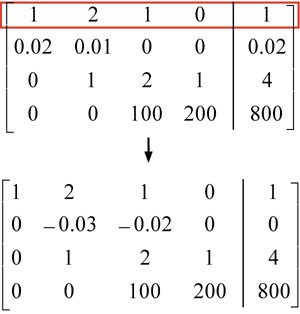Step 2: Find new pivot

Step 3: Switch rows (if necessary)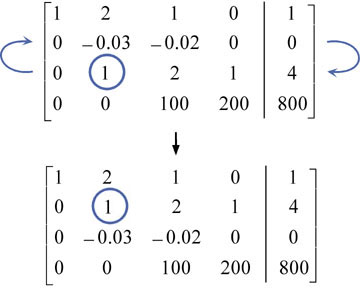Step 4: Gaussian Elimination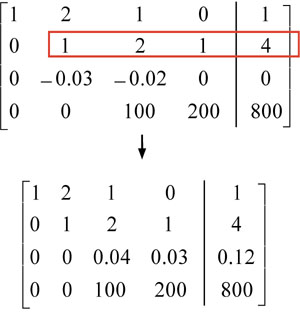Step 5: Find new pivot

Step 6: Switch rows (if necessary)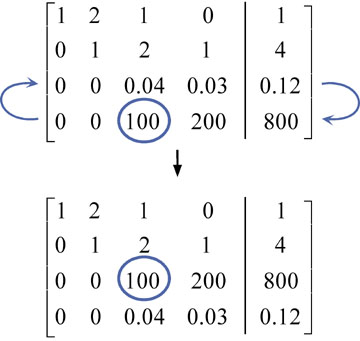Step 7: Gaussian Elimination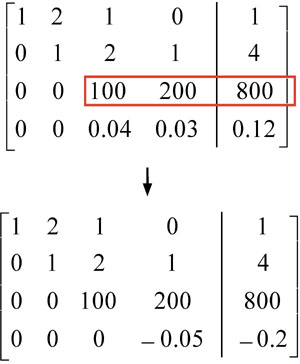Step 8: Back Substitute

-0.2x4 = -0.05;    x4 = 4

100x3 + 200x4 = 800;   x3 = 0

x2 + 2x3 + x4 =4;   x2 = 0

x1 + 2x2 + x3 = 1;   x1 = 1

Pivoting helps reduce rounding errors; you are less likely to add/subtract with very small number (or very large) numbers.